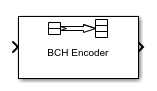# BCH Encoder

Create BCH code from binary vector data

• Library:
• Communications Toolbox / Error Detection and Correction / Block

•## Description

The BCH Encoder block creates a BCH code with message length K and codeword length (N – number of punctures).

If the encoder is processing multiple codewords per frame, then the same puncture pattern holds for all codewords. The input and output signal lengths are listed in Input and Output Signal Length in BCH Blocks.

See Tips for information about valid N values, valid (N,K) pairs, and error-correcting capabilities for a given BCH code.

## Ports

### Input

expand all

Message to encode, specified as a binary column vector input signal with an integer multiple of Message length, K elements or Shortened message length, S elements if the code is shortened. Each group of input elements represents one message word to encode. The input and output signal lengths are listed in Input and Output Signal Length in BCH Blocks.

Data Types: `single` | `double` | `int8` | `int16` | `int32` | `uint8` | `uint16` | `uint32` | `Boolean`

### Output

expand all

Encoded message, returned as a binary column vector. The encoded message is a BCH code with message length K and codeword length (N – number of punctures).

Data Types: `single` | `double` | `int8` | `int16` | `int32` | `uint8` | `uint16` | `uint32` | `Boolean`

For more information, see Supported Data Types.

## Parameters

expand all

Codeword length, specified as an integer of the form N = 2M–1, where M is an integer from 3 through 16. For more information, see Tips.

Message length, specified as an integer. The (`N`, `K`) pair must produce a narrow-sense BCH code.

Shortened message length, specified as an integer. When you specify this parameter, provide full-length N and K values to specify the (N, K) code that is shortened to an (NK+S, S) code.

#### Dependencies

To enable this parameter, select .

Generator polynomial, specified as one of the following:

• A polynomial character vector — For more information, see Representation of Polynomials in Communications Toolbox.

• A binary row vector that represents the coefficients of the generator polynomial in order of descending power.

• A binary Galois row vector that represents the coefficients of the generator polynomial in order of descending power.

Example: `'X^10 + X^8 + X^5 + X^4 + X^2 + X + 1'`, which is equivalent to `bchgenpoly(15,5)`

#### Dependencies

To enable this parameter, select .

Primitive polynomial in order of descending power. It is a polynomial of order M that defines the finite Galois field GF(2), specified as one of the following:

Example: `'X^4 + X + 1'`, which is the primitive polynomial used for a (15,5) code, ```ppoly = primpoly(4,'nodisplay'); int2bit(ppoly,ceil(log2(max(ppoly))))'```

#### Dependencies

To enable this parameter, select .

Select this parameter to disable generator polynomial check.

Each time a model initializes, the block performs a polynomial check. This check verifies that X N + 1 is divisible by the specified generator polynomial, where N represents the full codeword length. For larger codes, disabling the check speeds up the simulation process.

Tip

Always run the check at least once before disabling this feature.

#### Dependencies

To enable this parameter, select .

Puncture vector, specified as a binary column vector of length NK. Element indices with `1`s represent data symbol indices that pass through the block unaltered. Element indices with `0`s represent data symbol indices that get punctured, or removed, from the data stream. For more information, see Shortening, Puncturing, and Erasures.

Note

`1`s and `0`s have precisely opposite meanings for the puncture and erasure vectors. For an erasure vector, `1` means that the data symbol is to be replaced with an erasure symbol, and `0` means that the data symbol is passed through the block unaltered. This convention applies to both the encoder and the decoder.

#### Dependencies

To enable this parameter, select .

## Block Characteristics

 Data Types `Boolean` | `double` | `integer` | `single` Multidimensional Signals `no` Variable-Size Signals `no`

expand all

## Tips

• To generate the list of valid (`N`,`K`) pairs along with the corresponding values of the error-correction capability, run `bchnumerr`(`N`).

• Valid values for `N` = 2M–1, where M is an integer from 3 through 16. The maximum allowable value of `N` is 65,535.

## Algorithms

This block implements the algorithm, inputs, and outputs described in Algorithms for BCH and RS Errors-only Decoding.

 Clark, George C., Jr., and J. Bibb Cain. Error-Correction Coding for Digital Communications. New York: Plenum Press, 1981.